PodcastПрослушало
260

# Basic Vocabulary for Mathematics

LEVEL 2, 3, 4 |

I'm sure you know how to count to ten in English.
But what other vocabulary related to mathematics do you know?

Written and voiced by Mary Mironova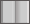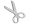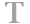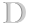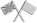Basic Vocabulary for Mathematics

Hi, guys!
This podcast is for those of you who need to know the basics of mathematics in English.

So, let's start out with addition.

When we add two numbers, we find their total, or sum. For example, two plus three equals five.
Plus is the sign we use for addition. The “equals” sign means that the left part of the line is the same as the right.

How much is thirty added to twenty five?
Yep, you're right! It's fifty five.

*  *  *  *  *

Subtraction is the opposite of addition.

When we subtract a number from another number, we take it away. For example, ten minus three is seven.

How much is fifteen minus four?
Sure, you're good at this! It's eleven.

*  *  *  *  *

When we multiply two numbers, we take one number the amount of times that equals the second number. If we multiply two by three, it means that we take two three times and add them. Two times three equals six. For multiplication, we often use this form:

[number] times [another number] equals [the result]”.

How much is four times nine?
Yay! It's 36. You're smart!

The numbers we get by multiplying small numbers (from 1 to 10 or 12) are shown in the multiplication table.
Do you know it by heart?

*  *  *  *  *

Division is the opposite of multiplication.

When we divide a number by another number, we find how many times the second number fits into the first number. For example, twelve divided by four is three.

How much is ten divided by two?
Yep, it's five.

*  *  *  *  *

If we raise a number to a power, it means that we multiply this number by itself as many times as shown in the power.
Three to the second power means that we multiply three by three. We can also say “three squared”.

Squared means the same as “raised to the second power”. If we raise five to the third power, it means that we need to multiply five by five by five again. It's five cubed. Cubed is like “raised to the third power”.
We can also say that we raise a number to the power of another number. For example, two raised to the power of six is sixty four.

So how much is ten to the second power?
It's one hundred!
How much is two to the fifth power?
It's 32.

*  *  *  *  *

If we take the square root of a number, it means that we need to find a number that gives us the number in the square root when multiplied by itself.
If we take the square root of twenty five, we get five. That means that if we multiply five by five we get 25.

What is the square root of 64?
Its 8!